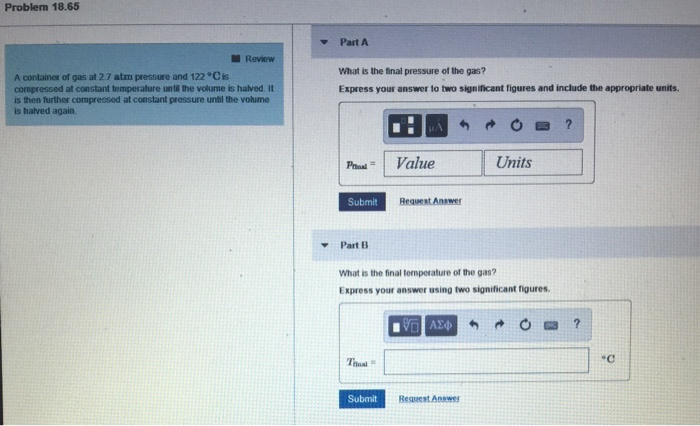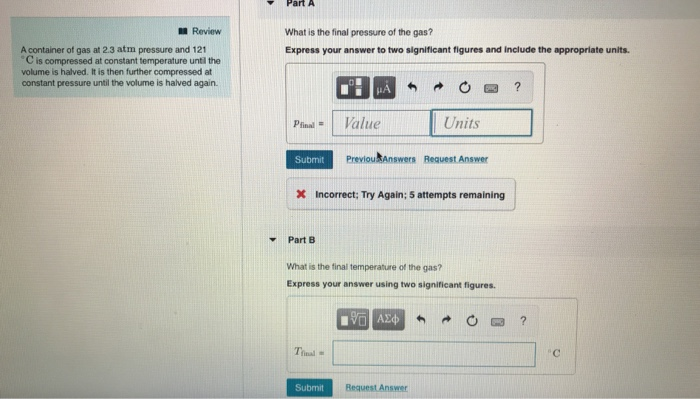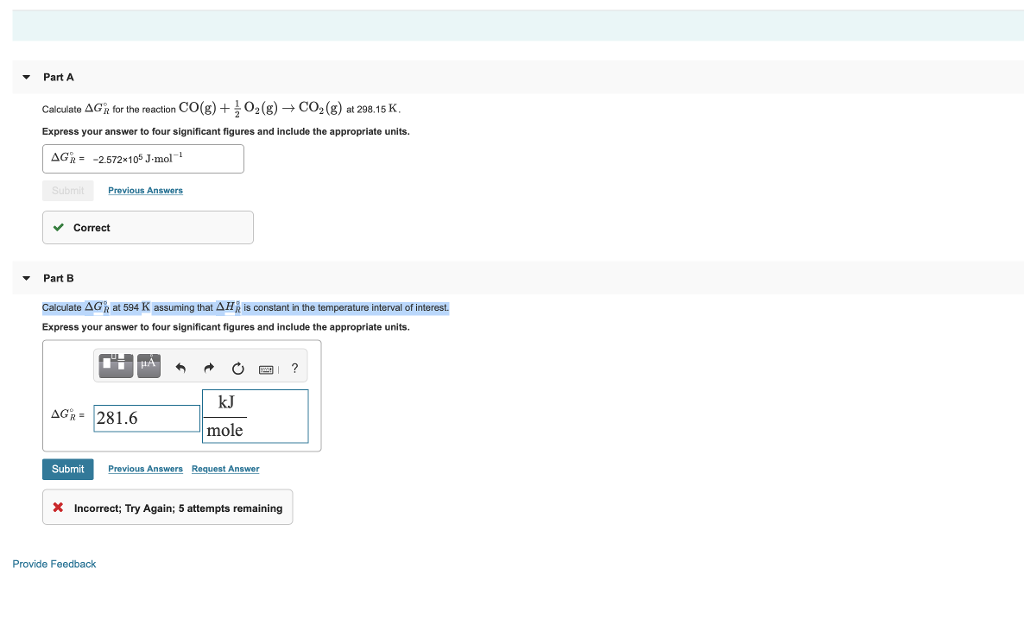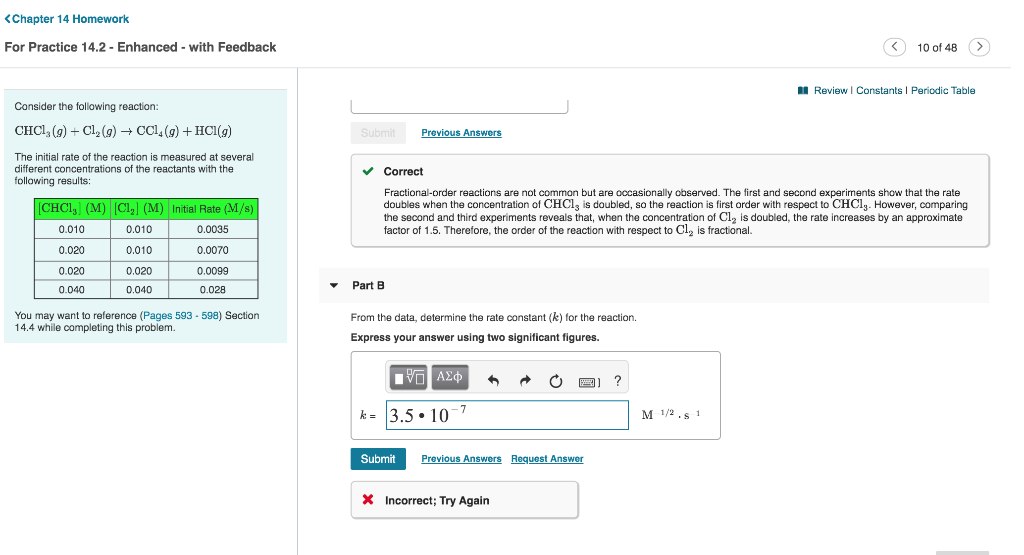Question

Determine the value of ΔSsurr at 325 K and assume constant pressure.
Express your answer to 3 significant figures in units of kJ mol-1 K-1.

4NH3(g) + 3O2(g) → 2N2(g) + 6H2O(g) ΔrH = -1267 kJ mol-1

∆Ssurr = -∆H/T

∆Hrxn = -1267 KJ/mol

T = 325 K

∆Ssurr = -(-1267)/325

= 3.90 KJ/mol.K

#### Earn Coins

Coins can be redeemed for fabulous gifts.

Similar Homework Help Questions
• ### Calculate ΔSsurr at the indicated temperature for a reaction. ΔH∘rxn= 126 kJ ; 66 K Express...

Calculate ΔSsurr at the indicated temperature for a reaction. ΔH∘rxn= 126 kJ ; 66 K Express your answer using two significant figures.

• ### For the reaction below, determine the equilibrium partial pressure (in atm) of H2 at 714.10 K...

For the reaction below, determine the equilibrium partial pressure (in atm) of H2 at 714.10 K if the initial pressure of HBr is 1.24 atm. Assume that ΔH and ΔS do not vary with temperature. Report your answer to three significant figures. LiH (s) + HBr (g) ⇌ LiBr (s) + H2 (g)   ΔHf° (kJ/mol)     S° (J mol-1 K-1) LiBr -351.20 74.30 H2 0.00 130.68 LiH -90.50 20.00 HBr -36.29 198.70

• ### Estimate the value of the equilibrium constant at 610 KK for each of the following reactions....

Estimate the value of the equilibrium constant at 610 KK for each of the following reactions. ΔG∘fΔGf∘ for BrCl(g)BrCl(g) is −−1.0 kJ/molkJ/mol. The standard molar entropy, S∘S∘, for BrCl(g)BrCl(g) is 240.0 J/mol⋅KJ/mol⋅K. Part A 2NO2(g)⇌N2O4(g).2NO2(g)⇌N2O4(g). ΔH∘fΔHf∘ for N2O4(g)N2O4(g) is 9.16 kJ/molkJ/mol. Express your answer using three significant figures. K= ??? Part B Br2(g)+Cl2(g)⇌2BrCl(g).Br2(g)+Cl2(g)⇌2BrCl(g). ΔH∘fΔHf∘ for BrCl(g)BrCl(g) is 14.6 kJ/molkJ/mol. Express your answer using three significant figures. K= ???

• ### A reaction has a rate constant of 1.21×10−4 s−1 at 25 ∘C and 0.226 s−1 at...

A reaction has a rate constant of 1.21×10−4 s−1 at 25 ∘C and 0.226 s−1 at 77 ∘C . Part A Determine the activation barrier for the reaction. Express your answer in units of kilojoules per mole and with 3 significant figures. Ea E a = nothing kJ/mol Request Answer Part B What is the value of the rate constant at 18 ∘C ? Express your answer in units of inverse seconds (s−1) and with 3 significant figures. k k...

• ### Problem 18.65 Part A Review What is the final pressure of the gas? Express your answer...Problem 18.65 Part A Review What is the final pressure of the gas? Express your answer to two significant figures and include the appropriate units A container of gas at 2.7 atm pressure and 122 Cis compressed at constant temperalture until the volume is halved. It is then further compressed at constant pressure until the volume is halved again P Value Units Submit Request Answer ▼Part13 What is the final temperature of the gas? Express your answer using two significant...

• ### PartA Review What is the final pressure of the gas? Express your answer to two significant...PartA Review What is the final pressure of the gas? Express your answer to two significant figures and include the appropriate units. A container of gas at 2.3 atm pressure and 121 C is compressed at constant temperature until the volume is halved. It is then further compressed at constant pressure until the volume is halved again. pfinal- 1 Value Units Submit Previou Answers Request Answer X Incorrect; Try Again; 5 attempts remaining Part B What is the final temperature...

• ### 1) ?H?rxn= 129 kJ , ?S?rxn=? 259 J/K , T= 290 K . Express your answer...

1) ?H?rxn= 129 kJ , ?S?rxn=? 259 J/K , T= 290 K . Express your answer using three significant figures. ?Suniv= ? (K/J) 2) ?H?rxn=? 129 kJ , ?S?rxn= 259 J/K , T= 290 K . Express your answer using three significant figures. ?Suniv= ? (K/J) 3) ?H?rxn=? 129 kJ , ?S?rxn=? 259 J/K , T= 290 K . Express your answer using three significant figures. ?Suniv= ? (K/J) 4) ?H?rxn=? 129 kJ , ?S?rxn=? 259 J/K , T= 553...

• ### Calculate ΔG∘R at 594 K assuming that ΔH∘R is constant in the temperature interval of interest....Calculate ΔG∘R at 594 K assuming that ΔH∘R is constant in the temperature interval of interest. Part A Calculate ΔGe for the reaction Express your answer to four significant figures and include the appropriate units. CO(g) + ,02 (g) CO2 (g) at 298.15 K ΔGR: -2572x105 J-mol 1 Previous Answers Correct Part B Calculate ΔGk at 594 K assuming that ΔΗ¡ is constant in the temperature interval of interest Express your answer to four significant figures and include the appropriate...

• ### One mole of O2(g), with CV,m=2.5R,  is expanded adiabatically from 301 K and 4.00 bar to 1.60...

One mole of O2(g), with CV,m=2.5R,  is expanded adiabatically from 301 K and 4.00 bar to 1.60 bar against a constant external pressure equal to the final pressure. Calculate q, w, ΔU, ΔH, ΔS and ΔSsurr. Enter your answers in the specified units with three or more significant figures . Do not include units as part of your answer.    q = ____________ J    w = ____________ J ΔU = ____________ J ΔH = ____________ J ΔS = ____________ J mol-1 K-1...

• ### From the data, determine the rate constant (k) for the reaction. Express your answer using two...From the data, determine the rate constant (k) for the reaction. Express your answer using two significant figures. <Chapter 14 Homework For Practice 14.2 - Enhanced - with Feedback 10 of 48 Review I Constants Periodic Table Consider the following reaction: CHCl (g)Cl(g) -CCl (g) +HCl(g) Submit Previous Answers The initial rate of the reaction is measured at several different concentrations of the reactants with the Correct following results Fractional-order reactions are not comman but are occasionally observed. The first...